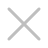• 中信红木
• 国寿红木
• 巧夺天工
• 泰和园
• 红古轩
• 明堂红木
• 卓木王
• 居典红木
• 恒达木业
• 新明红木
• 国方家居
• 雅宋红木
• 鲁班木艺
• 古森红木
• 双洋红木
• 御乾堂
• 国祥红木
• 顺泰轩
• 华行红木
• 闲适红木
• 致上家居
• 雅晟檀一
• 兴成阅见
• 万事红
• 印巷森刻
• 地天泰国风
• 志成红木
• 雅典红木
• 老周家居
• 戴为红木
• 华厦·大不同
• 万家宜红木
• 清韵红木
• 伟明轩
• 创辉红木
• 雅仕轩
• 红桥红
• 大家之家
• 东成红木
• 鸿运堂
• 富宝轩红木
• 祥盛居
• 苏阳红
• 区氏臻品
• 御华轩
• 旭东红木
• 一品居、宏达豪典
• 明清居
• 缘人缘
• 永恒居
• 年年红
• 华夏一品
• 越府红木
• 万盛宇红木
• 永华红木
• 鸿庭轩红木
• 本木上造
• 雅居阁
• 良辰轩
• 红天下
• 九龙堂
• 广作红木研究院
• 龙珍阁红木
• 东方之信红木
• 春风
• 林木轩
• 古雨轩
• 云林堂
• 汉华瑰宝
• 木缘红木
• 水雨轩功夫茶台
• 盛世和艺轩
• 旭东红木
• 大清御品
• 东方荟
• 怀古
• 宝鹰红木
• 铭华
• 天工隽品
• 唐明居
• 振宇红木
• 大汇堂红木家具
• 雍王府红木
• 海龙红木
• 泰森红木
• 华木苑红木
• 林达宏红木
• 中港红木
• 雍庭红木
• 大户人家红木
• 文宝轩红木
• 陈王阁
• 南枝红红木
• 孔连红木
• 华钦家具
• 名扬红木
• 大连中海家居
• 汉府家具
• 大家风范
• 福居阁
• 秀饰坊
• 祥瑞坊红木
• 伟世鸿泰家具
• 常记家居
• 汉韵宝鼎家具
• 玖月红木
• 盘谷红木
• 沽之大匠
• 無名红木
• 波记家具
• 长丰
• 红宝轩
• 自在堂红木
• 钧福楼
• 伍氏大观园
• 如金红木
• 红木屋
• 通天红红木
• 东邦红木
• 乾珑堂红木
• 微凹传奇
• 拾贰空间
• 东方宝鼎红木
• 龙博士
• 盛世周木匠
• 太和木作
• 世珀
• 五华产业园
• 博大家具
• 匠王红木
• 卢家艺林
• 大成尚品
• 中唐红木城
• 大东家红木
• 名典红木
• 传天匠
• 香山桃园
• 森木家具
• 长汇红木
• 名作红木
• 梦响红木
• 广工坊红木
• 镜观家居
• 祥晟隆红木
• 鸿韵红木
• 德成红木
• 干龙轩红木
• 朗州上乘
• 环宇一品
• 致远家具
• 冠森红木
• 翰文红木
• 墨+
• 荣燊堂红木
• 天勤红木
• 轩禾殿
• 盛世堂红木
• 欧晨卫浴
• 金品世家
• 榫卯大师
• 金隆红木
• 紫福堂
• 豪族旺家
• 禾木尚品
• 明尊古典
• 古木印象
• 沁园居红木
• 0-10000
• 10001-20000
• 20001-40000
• 40001-80000
• 80000以上
• 面议
• 大果紫檀(缅甸花梨)
• 阔叶黄檀(印尼黑酸枝)
• 刺猬紫檀
• 交趾黄檀(大红酸枝)
• 巴里黄檀(花枝)
• 降香黄檀(海南黄花梨)
• 檀香紫檀(小叶紫檀)
• 奥氏黄檀(白酸枝)
• 微凹黄檀(红酸枝)
• 绒毛黄檀(红酸枝)
• 东非黑黄檀(紫光檀)
• 卢氏黑黄檀(大叶紫檀)
• 越南黄花梨
• 染料紫檀(非洲血檀)
• 鸡翅木
• 古夷苏木(巴花)
• 红铁木豆(小叶红檀)
• 螺穗木(非洲檀香)
• 沉贵宝(东非酸枝)
• 红贵宝(非洲酸枝)
• 小巴花(东非香花梨)
• 柬埔寨黑酸枝
• 安哥拉紫檀(红高棉)
• 非洲紫檀(红花梨)
• 其他名贵硬木
• 其他工艺品
• 其他
• 书房系列
• 客厅系列
• 餐厅系列
• 茶室系列
• 套房系列
• 其它系列
• 新古典
• 新中式
• 明清古典
• 欧式古典
• 其他风格
• 香几类
• 屏风类
• 柜架类
• 宝座类
• 凳墩类
• 坐椅类
• 床榻类
• 桌案类
• 沙发类

• 中信红木
• 国寿红木
• 巧夺天工
• 泰和园
• 红古轩
• 明堂红木
• 卓木王
• 居典红木
• 恒达木业
• 新明红木
• 国方家居
• 雅宋红木
• 鲁班木艺
• 古森红木
• 双洋红木
• 御乾堂
• 国祥红木
• 顺泰轩
• 华行红木
• 闲适红木
• 致上家居
• 雅晟檀一
• 兴成阅见
• 万事红
• 印巷森刻
• 地天泰国风
• 志成红木
• 雅典红木
• 老周家居
• 戴为红木
• 华厦·大不同
• 万家宜红木
• 清韵红木
• 伟明轩
• 创辉红木
• 雅仕轩
• 红桥红
• 大家之家
• 东成红木
• 鸿运堂
• 富宝轩红木
• 祥盛居
• 苏阳红
• 区氏臻品
• 御华轩
• 旭东红木
• 一品居、宏达豪典
• 明清居
• 缘人缘
• 永恒居
• 年年红
• 华夏一品
• 越府红木
• 万盛宇红木
• 永华红木
• 鸿庭轩红木
• 本木上造
• 雅居阁
• 良辰轩
• 红天下
• 九龙堂
• 广作红木研究院
• 龙珍阁红木
• 东方之信红木
• 春风
• 林木轩
• 古雨轩
• 云林堂
• 汉华瑰宝
• 木缘红木
• 水雨轩功夫茶台
• 盛世和艺轩
• 旭东红木
• 大清御品
• 东方荟
• 怀古
• 宝鹰红木
• 铭华
• 天工隽品
• 唐明居
• 振宇红木
• 大汇堂红木家具
• 雍王府红木
• 海龙红木
• 泰森红木
• 华木苑红木
• 林达宏红木
• 中港红木
• 雍庭红木
• 大户人家红木
• 文宝轩红木
• 陈王阁
• 南枝红红木
• 孔连红木
• 华钦家具
• 名扬红木
• 大连中海家居
• 汉府家具
• 大家风范
• 福居阁
• 秀饰坊
• 祥瑞坊红木
• 伟世鸿泰家具
• 常记家居
• 汉韵宝鼎家具
• 玖月红木
• 盘谷红木
• 沽之大匠
• 無名红木
• 波记家具
• 长丰
• 红宝轩
• 自在堂红木
• 钧福楼
• 伍氏大观园
• 如金红木
• 红木屋
• 通天红红木
• 东邦红木
• 乾珑堂红木
• 微凹传奇
• 拾贰空间
• 东方宝鼎红木
• 龙博士
• 盛世周木匠
• 太和木作
• 世珀
• 五华产业园
• 博大家具
• 匠王红木
• 卢家艺林
• 大成尚品
• 中唐红木城
• 大东家红木
• 名典红木
• 传天匠
• 香山桃园
• 森木家具
• 长汇红木
• 名作红木
• 梦响红木
• 广工坊红木
• 镜观家居
• 祥晟隆红木
• 鸿韵红木
• 德成红木
• 干龙轩红木
• 朗州上乘
• 环宇一品
• 致远家具
• 冠森红木
• 翰文红木
• 墨+
• 荣燊堂红木
• 天勤红木
• 轩禾殿
• 盛世堂红木
• 欧晨卫浴
• 金品世家
• 榫卯大师
• 金隆红木
• 紫福堂
• 豪族旺家
• 禾木尚品
• 明尊古典
• 古木印象
• 沁园居红木

• 0-10000
• 10001-20000
• 20001-40000
• 40001-80000
• 80000以上
• 面议

• 大果紫檀(缅甸花梨)
• 阔叶黄檀(印尼黑酸枝)
• 刺猬紫檀
• 交趾黄檀(大红酸枝)
• 巴里黄檀(花枝)
• 降香黄檀(海南黄花梨)
• 檀香紫檀(小叶紫檀)
• 奥氏黄檀(白酸枝)
• 微凹黄檀(红酸枝)
• 绒毛黄檀(红酸枝)
• 东非黑黄檀(紫光檀)
• 卢氏黑黄檀(大叶紫檀)
• 越南黄花梨
• 染料紫檀(非洲血檀)
• 鸡翅木
• 古夷苏木(巴花)
• 红铁木豆(小叶红檀)
• 螺穗木(非洲檀香)
• 沉贵宝(东非酸枝)
• 红贵宝(非洲酸枝)
• 小巴花(东非香花梨)
• 柬埔寨黑酸枝
• 安哥拉紫檀(红高棉)
• 非洲紫檀(红花梨)
• 其他名贵硬木
• 其他工艺品
• 其他

• 书房系列
• 客厅系列
• 餐厅系列
• 茶室系列
• 套房系列
• 其它系列

• 新古典
• 新中式
• 明清古典
• 欧式古典
• 其他风格

• 香几类
• 屏风类
• 柜架类
• 宝座类
• 凳墩类
• 坐椅类
• 床榻类
• 桌案类
• 沙发类